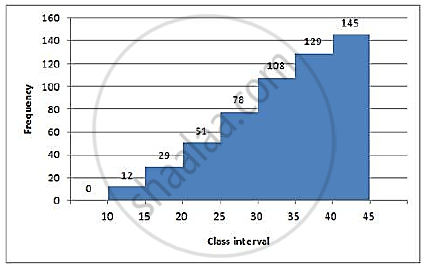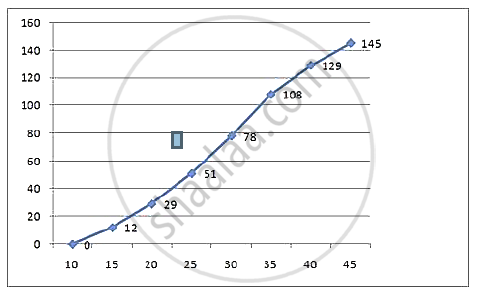# (A) from the Distribution, Given Above, Construct a Frequency Table. (B) Use the Table Obtained in Part (A) to Draw: (I) a Histogram, (Ii) an Ogive - Mathematics

Sum
 Class mark 12.5 17.5 22.5 27.5 32.5 32.5 42.5 Frequency 12 17 22 27 30 21 16

(a) From the distribution, given above, construct a frequency table.
(b) Use the table obtained in part (a) to draw: (i) a histogram, (ii) an ogive

#### Solution

(a) Difference in consecutive class marks = 17.5 − 12.5 = 5
⇒ first class interval will be 10-15 and so on.

 Class Mark Class Interval Frequency c.f. 12.5 10-15 12 12 17.5 15-20 17 29 22.5 20-25 22 51 27.5 25-30 27 78 32.5 30-35 30 108 37.5 35-40 21 129 42.5 40-45 16 145

Total = 145(b) Now plot the points (15,12), (20,29), (25,51), (30,78), (35,108), (40,129), (45,145) and join them to obtain an ogive.Concept: Finding the Median, upper quartile, lower quartile from the Ogive
Is there an error in this question or solution?

#### APPEARS IN

Selina Concise Maths Class 10 ICSE
Chapter 23 Graphical Representation
Exercise 23 | Q 6 | Page 349